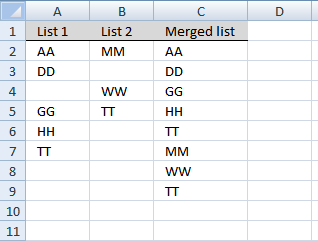Author: Oscar Cronquist Article last updated on February 16, 2018

### Question:

This article is terrific. Thanks so much for posting this solution!

I do have one question:

Let's say my "List 1" is auto updated and the number of entries in this list will fluctuate. Since the number of entries fluctuates, I would like to select a larger range than I actually have data in currently. The issue is when I make my "List 1" larger than the number of entries, the rows that don't currently have data in them, show up on my combined list as zeros.

So my question is, is there a way to adjust the formula so that when it looks at "List 1" for example, it skips over blank cells and continues to combine the list with "List 2".

(You can find the question here: Merge two columns into one list in excel)

### Answer:

The array formula below removes blank cells. Another method is to use dynamic named ranges.### Array formula in C2:

=IFERROR(INDEX(List1, SMALL(IF(ISBLANK(List1), "", ROW(List1)-MIN(ROW(List1))+1), ROW(A1))), IFERROR(INDEX(List2, SMALL(IF(ISBLANK(List2), "", ROW(List2)-MIN(ROW(List2))+1), ROW(A1)-SUMPRODUCT(--NOT((ISBLANK(List1)))))), ""))

Recommended post:

Combine cell ranges ignore blank cells

The image above demonstrates a user defined function that merges up to 255 cell ranges and removes blanks. I will also […]

This is an array formula, here is how to enter it. Type the formula in cell C2, press and hold CTRL + SHIFT simultaneously. Press Enter once. Release all keys. If you did it correctly, you now have curly brackets before and after the formula.

Copy cell C2 and paste it to cells below, as far as needed.

This example merges two columns into one column using an array formula. If you are looking for merging two data lists with criteria, check this post: Merge lists with criteria

### Named ranges

List1 (A2:A7)
List2 (B2:B5)

Recommended article:

Merge three columns into one list

The above image demonstrates a formula that adds values in three different columns into one column. Formula in H2: =IFERROR(INDEX(\$B\$3:\$B\$7, […]

### Explaining array formula in cell C8

Step 1 - Understand relative and absolute cell referencing

In cell C2 the formula is:

=IFERROR(INDEX(List1, SMALL(IF(ISBLANK(List1), "", ROW(List1)-MIN(ROW(List1))+1), ROW(A1))), IFERROR(INDEX(List2, SMALL(IF(ISBLANK(List2), "", ROW(List2)-MIN(ROW(List2))+1), ROW(A1)-SUMPRODUCT(--NOT((ISBLANK(List1)))))), ""))

In cell C8 the formula is:

=IFERROR(INDEX(List1, SMALL(IF(ISBLANK(List1), "", ROW(List1)-MIN(ROW(List1))+1), ROW(A7))), IFERROR(INDEX(List2, SMALL(IF(ISBLANK(List2), "", ROW(List2)-MIN(ROW(List2))+1), ROW(A7)-SUMPRODUCT(--NOT((ISBLANK(List1)))))), ""))

How to use absolute and relative references

What is a reference in Excel? Excel has an A1 reference style meaning columns are named letters A to XFD […]

Step 1 - Find cells containing a value in List 1 and return row numbers in an array

=IFERROR(INDEX(List1, SMALL(IF(ISBLANK(List1), "", ROW(List1)-MIN(ROW(List1))+1), ROW(A7))), IFERROR(INDEX(List2, SMALL(IF(ISBLANK(List2), "", ROW(List2)-MIN(ROW(List2))+1), ROW(A7)-SUMPRODUCT(--NOT((ISBLANK(List1)))))), ""))

IF(ISBLANK(List1), "", ROW(List1)-MIN(ROW(List1))+1)

becomes

IF(ISBLANK({"AA";"DD";"";"GG";"HH";"TT"}), "", ROW(A2:A7)-MIN(ROW(A2:A7))+1)

becomes

IF({FALSE;FALSE;TRUE;FALSE;FALSE;FALSE}, "", {2;3;4;5;6;7}-MIN({2;3;4;5;6;7})+1)

becomes

IF({FALSE;FALSE;TRUE;FALSE;FALSE;FALSE}, "", {2;3;4;5;6;7}-2+1)

becomes

IF({FALSE;FALSE;TRUE;FALSE;FALSE;FALSE}, "", {1;2;3;4;5;6})

and returns

{1;2;"";4;5;6}

How to use the IF function

Checks if a logical expression is met. Returns a specific value if TRUE and another specific value if FALSE.

Step 2 - Return the k-th smallest row number

=IFERROR(INDEX(List1, SMALL(IF(ISBLANK(List1), "", ROW(List1)-MIN(ROW(List1))+1), ROW(A7))), IFERROR(INDEX(List2, SMALL(IF(ISBLANK(List2), "", ROW(List2)-MIN(ROW(List2))+1), ROW(A7)-SUMPRODUCT(--NOT((ISBLANK(List1)))))), ""))

SMALL(array,k) Returns the k-th smallest number in this data set.

SMALL(IF(ISBLANK(List1), "", ROW(List1)-MIN(ROW(List1))+1), ROW(A7))

becomes

SMALL({1;2;"";4;5;6}, 8 )

and returns #NUM

How to use the SMALL function

The SMALL function lets you extract a number in a cell range based on how small it is compared to the other numbers in the group.

Step 3 - Return a value of the cell at the intersection of a particular row and column

=IFERROR(INDEX(List1, SMALL(IF(ISBLANK(List1), "", ROW(List1)-MIN(ROW(List1))+1), ROW(A7))), IFERROR(INDEX(List2, SMALL(IF(ISBLANK(List2), "", ROW(List2)-MIN(ROW(List2))+1), ROW(A7)-SUMPRODUCT(--NOT((ISBLANK(List1)))))), ""))

INDEX(array,row_num,[column_num]) returns a value or reference of the cell at the intersection of a particular row and column, in a given range

INDEX(List1, SMALL(IF(ISBLANK(List1), "", ROW(List1)-MIN(ROW(List1))+1), ROW(A7)))

becomes

INDEX(List1, #NUM)

and returns

#NUM

How to use the INDEX function

Gets a value in a specific cell range based on a row and column number.

Step 4 - Check if formula returns an error

=IFERROR(INDEX(List1, SMALL(IF(ISBLANK(List1), "", ROW(List1)-MIN(ROW(List1))+1), ROW(A7))), IFERROR(INDEX(List2, SMALL(IF(ISBLANK(List2), "", ROW(List2)-MIN(ROW(List2))+1), ROW(A7)-SUMPRODUCT(--NOT((ISBLANK(List1)))))), ""))

becomes

=IFERROR(#NUM, IFERROR(INDEX(List2, SMALL(IF(ISBLANK(List2), "", ROW(List2)-MIN(ROW(List2))+1), ROW(A7)-SUMPRODUCT(--NOT((ISBLANK(List1)))))), ""))

becomes

IFERROR(INDEX(List2, SMALL(IF(ISBLANK(List2), "", ROW(List2)-MIN(ROW(List2))+1), ROW(A7)-SUMPRODUCT(--NOT((ISBLANK(List1)))))), "")

How to use the IFERROR function

The IFERROR function lets you catch most errors in Excel formulas. It was introduced in Excel 2007. In previous Excel […]

Step 5 - Find cells containing a value in List 2

IFERROR(INDEX(List2, SMALL(IF(ISBLANK(List2), "", ROW(List2)-MIN(ROW(List2))+1), ROW(A7)-SUMPRODUCT(--NOT((ISBLANK(List1)))))), "")

IF(ISBLANK(List2), "", ROW(List2)-MIN(ROW(List2))+1)

becomes

IF(ISBLANK({"MM";"";"WW";"TT"}), "", {2;3;4;5}-MIN({2;3;4;5})+1)

becomes

IF({FALSE;TRUE;FALSE;FALSE}), "", {1;2;3;4})

and returns

{1;"";3;4}

Step 6 - Return the k-th smallest row number

IFERROR(INDEX(List2, SMALL(IF(ISBLANK(List2), "", ROW(List2)-MIN(ROW(List2))+1), ROW(A8)-SUMPRODUCT(--NOT((ISBLANK(List1)))))), "")

SMALL(array,k) Returns the k-th smallest number in this data set.

SMALL(IF(ISBLANK(List2), "", ROW(List2)-MIN(ROW(List2))+1), ROW(A8)-SUMPRODUCT(--NOT((ISBLANK(List1)))))

becomes

SMALL({1;"";3;4}, ROW(A7)-SUMPRODUCT(--NOT((ISBLANK(List1)))))

becomes

SMALL({1;"";3;4}, 7-SUMPRODUCT(--NOT((ISBLANK({"AA";"DD";"";"GG";"HH";"TT"})))))

becomes

SMALL({1;"";3;4}, 7-SUMPRODUCT(--NOT({FALSE;FALSE;TRUE;FALSE;FALSE;FALSE})))

becomes

SMALL({1;"";3;4}, 7-SUMPRODUCT(--{TRUE;TRUE;FALSE;TRUE;TRUE;TRUE}))

becomes

SMALL({1;"";3;4}, 7-SUMPRODUCT({1;1;0;1;1;1})

becomes

SMALL({1;"";3;4}, 7-5)

becomes

SMALL({1;"";3;4}, 2)

and returns 3.

Step 6 - Return a value of the cell at the intersection of a particular row and column

IFERROR(INDEX(List2, SMALL(IF(ISBLANK(List2), "", ROW(List2)-MIN(ROW(List2))+1), ROW(A8)-SUMPRODUCT(--NOT((ISBLANK(List1)))))), "")

becomes

IFERROR(INDEX(List2, 3), "")

becomes

IFERROR(INDEX({"MM";0;"WW";"TT"}, 3), "")

becomes

IFERROR("WW", "")

and returns WW.

Download excel sample file for this tutorial.
merge-two-columns with blanks.xlsx
(Excel 2007 Workbook *.xlsx)

### Functions in this article:

ISBLANK(value)
Checks whether a reference is to an empty cell and returns TRUE or FALSE

MIN(number1,[number2])
Returns the smallest number in a set of values. Ignores logical values and text

How to use the ROW function

The ROW function calculates the row number of a cell reference. Excel Function Syntax ROW(reference) Arguments reference Optional. A reference […]

How to use the SUMPRODUCT function

The SUMPRODUCT function calculates the product of corresponding values and then returns the sum of each multiplication.

NOT(logical)
Changes FALSE to TRUE, or TRUE to FALSE